Type
Solution Manual
Book Title
Fundamentals of Investments: Valuation and Management 8th Edition
ISBN 13
978-1259720697

### 978-1259720697 Chapter 12 Solution Manual

January 2, 2020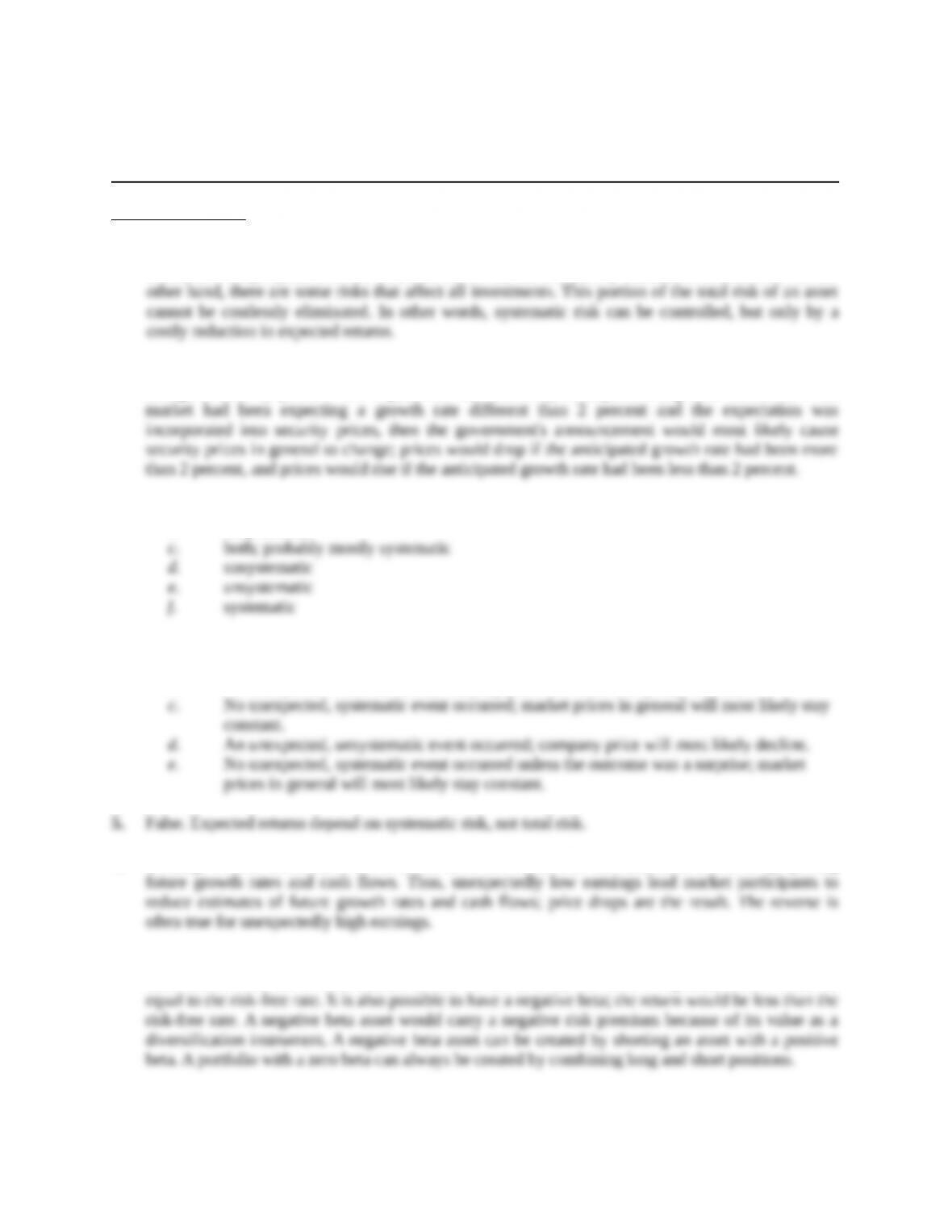Chapter 12
Return, Risk, and the Security Market Line
Concept Questions
1. Some of the risk in holding any asset is unique to the asset in question. By investing in a variety of
assets, this unique portion of the total risk can be almost completely eliminated at little cost. On the
2. If the market expected the growth rate in the coming year to be 2 percent, then there would be no
change in security prices if this expectation had been fully anticipated and priced. However, if the
3. a. systematic
b. unsystematic
4. a. An unexpected, systematic event occurred; market prices in general will most likely
decline.
b. No unexpected event occurred; company price will most likely stay constant.
6. Earnings contain information about recent sales and costs. This information is useful for projecting
7. Yes. It is possible, in theory, for a risky asset to have a beta of zero. Such an asset’s return is simply
uncorrelated with the overall market. Based on the CAPM, this asset’s expected return would be
Education.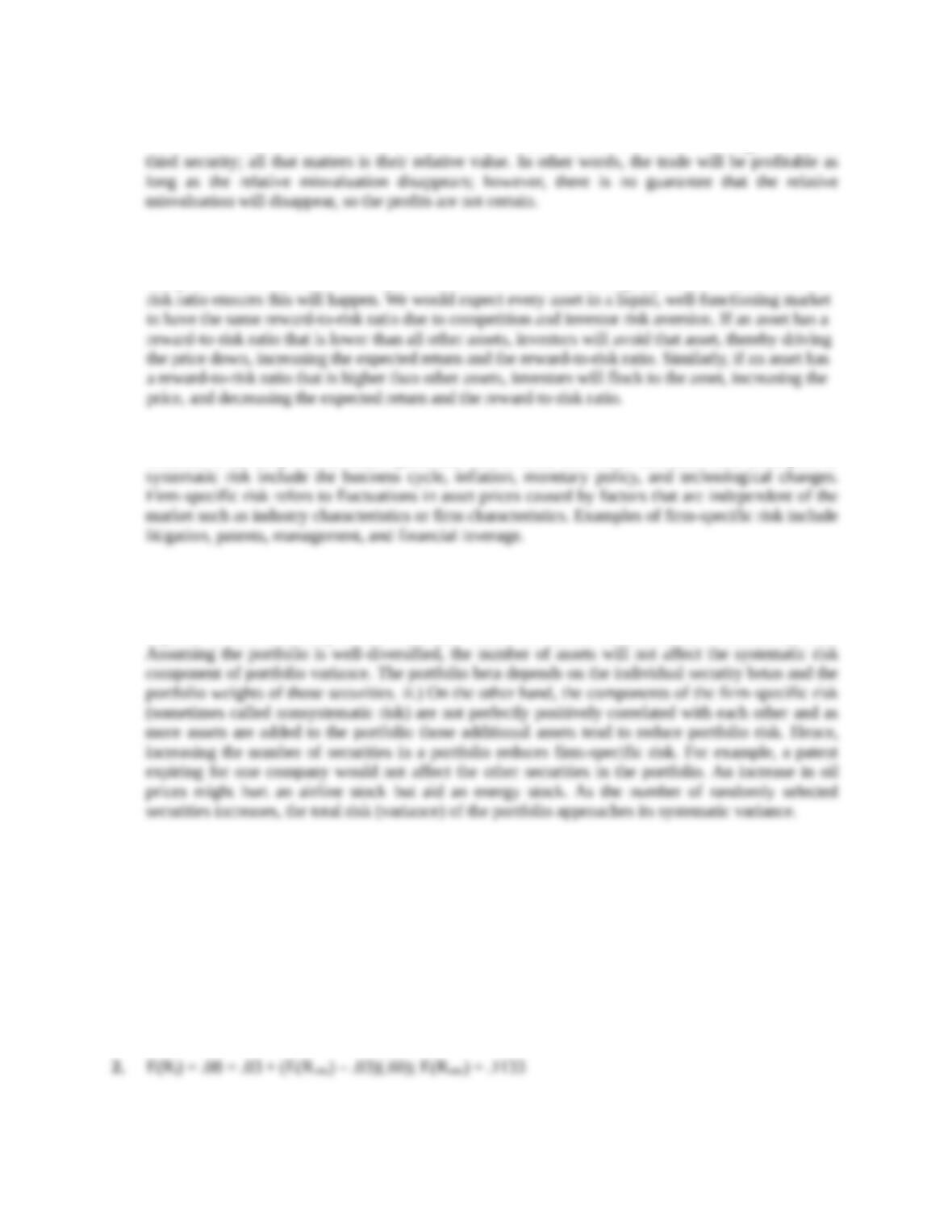8. The rule is always “buy low, sell high.” In this case, we buy the undervalued asset and sell (short) the
overvalued one. It does not matter whether the two securities are misvalued with regard to some
9. If every asset has the same reward-to-risk ratio, the implication is that every asset provides the same
risk premium for each unit of risk. In other words, the only way to increase your return (reward) is to
accept more risk. Investors will only take more risk if the reward is higher, and a constant reward-to-
10. a. Systematic risk refers to fluctuations in asset prices caused by macroeconomic factors that are
common to all risky assets; hence systematic risk is often referred to as market risk. Examples of
b. Trudy should explain to the client that picking only the top five best ideas would most likely result
in the client holding a much more risky portfolio. The total risk of the portfolio, or portfolio
variance, is the combination of systematic risk and firm-specific risk. i.) The systematic component
depends on the sensitivity of the individual assets to market movements as measured by beta.
Solutions to Questions and Problems
NOTE: All end of chapter problems were solved using a spreadsheet. Many problems require multiple
steps. Due to space and readability constraints, when these intermediate steps are included in this
solutions manual, rounding may appear to have occurred. However, the final answer for each problem is
found without rounding during any step in the problem.
Core Questions
1. E(Ri) = .132 = .035 + .075i ; i = 1.29
Education.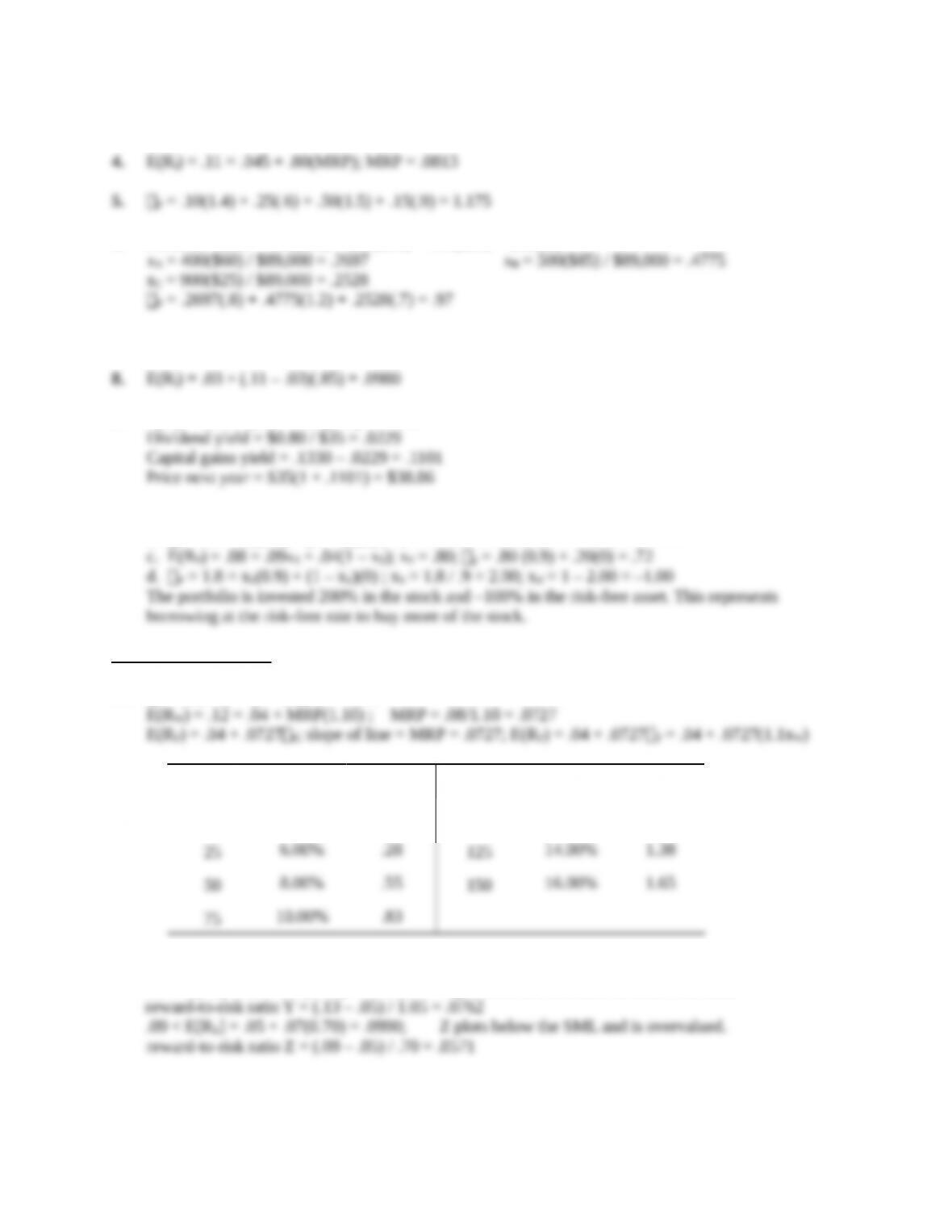3. E(Ri) = .12 = Rf + (.10 – Rf)(1.40); Rf = .05
6. Portfolio value = 400(\$60) + 500(\$85) + 900(\$25) = \$89,000
7. P = 1.0 = 1/3(0) + 1/3(1.20) + 1/3(X) ; X = 1.80
9. E(Ri) = .055 + (.12 – .055)(1.2) = .1330
10. a. E(RP) = (.09 + .04) / 2 = .0650
b. P = 0.5 = xS(0.9) + (1 – xS)(0) ; xS = .5 / .9 = .5556 ; xrf = 1 – .5556 = .4444
Intermediate Questions
11. P = xW(1.1) + (1 – xW)(0) = 1.1xW
xWE[rp]pxWE[rp]p
0% 4.00% .00 100% 12.00% 1.10
12. E[Rii] = .05 + .07i
.13 > E[RY] = .05 + .07(1.05) = .1235; Y plots above the SML and is undervalued.
13. [.13 – Rf]/1.05 = [.09 – Rf] / 0.70 ; Rf = .01
Education.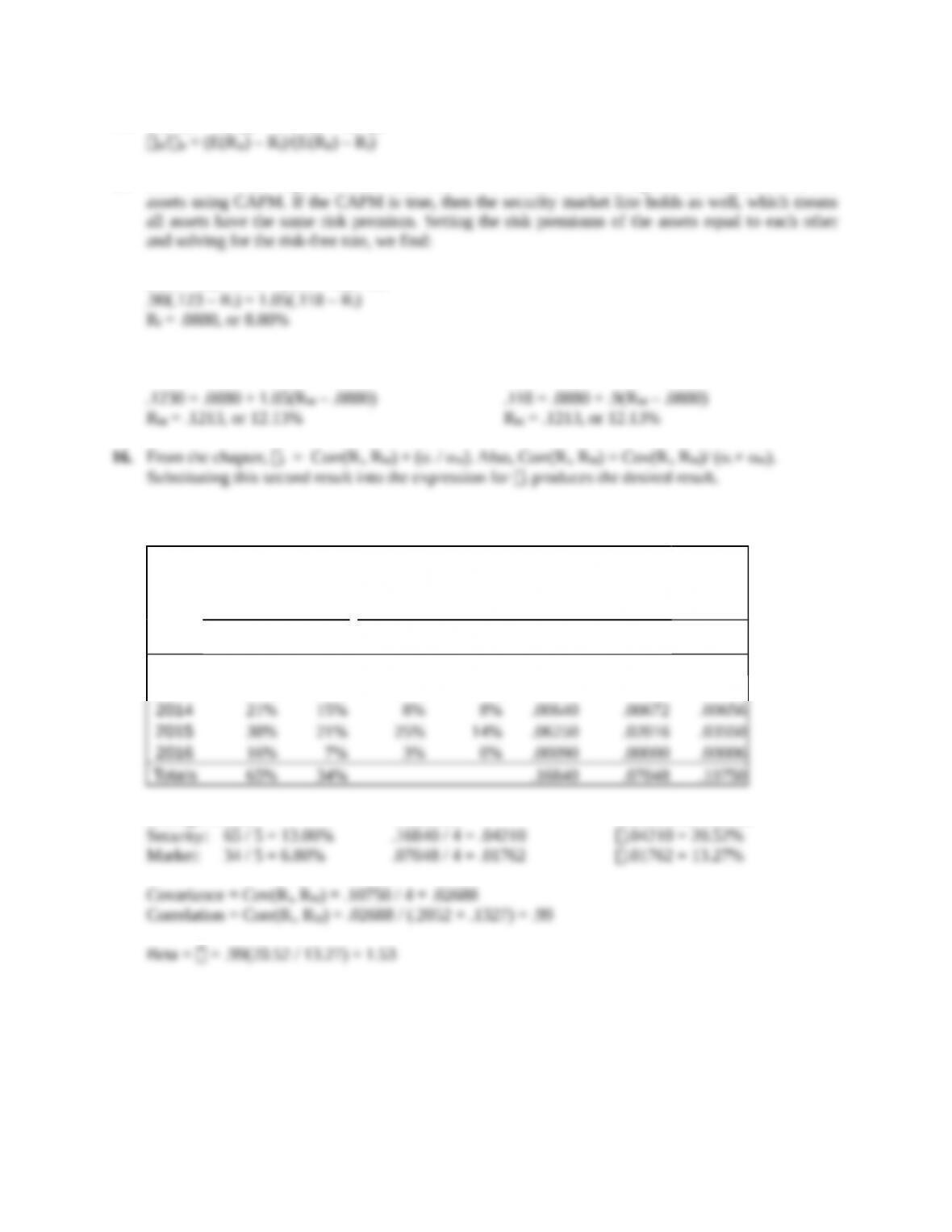14. (E(RA) – Rf)/ A = (E(RB) – Rf)/ B
15. Here we have the expected return and beta for two assets. We can express the returns of the two
(.123 – Rf) / 1.05 = (.118 – Rf) / .90
Now using CAPM to find the expected return on the market with both stocks, we find:
17. The relevant calculations can be summarized as follows:
Returns Return deviations Squared deviations
Product
of
deviations
Year Security Market Security Market Security Market
2012 8% 5% -5% -2% .00250 .00032 .00090
2013 -18% -14% -31% -21% .09610 .04326 .06448
Average returns: Variances: Standard deviations:
Education.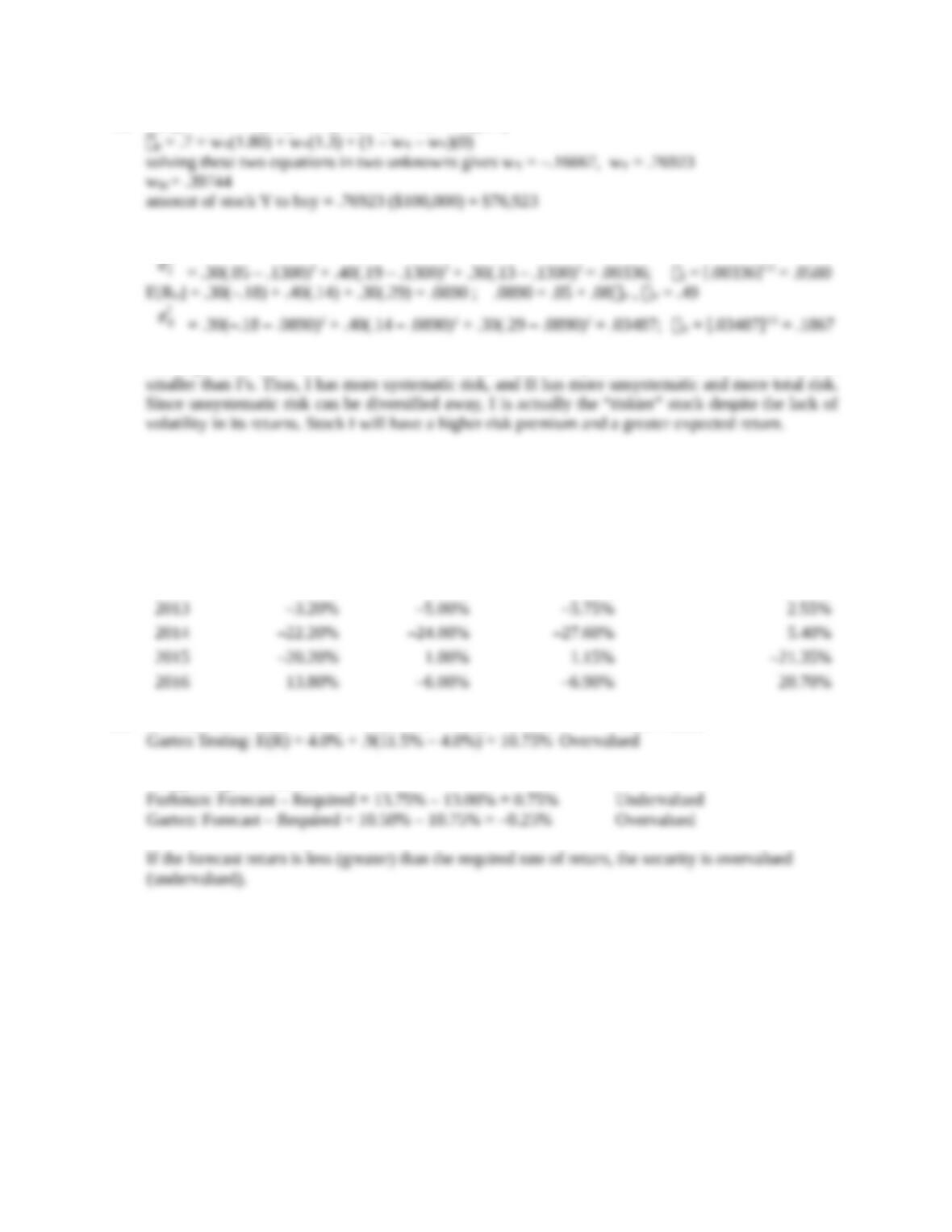18. E[Rp] = .13 = wX(.31) + wY(.20) + (1 – wX – wY)(.07)
19. E[RI] = .30(.05) + .40(.19) + .30(.13) = .1300 ; .1300 = .05 + .08I , I = 1.00
σI
2
σII
2
Although stock II has more total risk than I, it has much less systematic risk, since its beta is much
20. E(R) = .05 + 1.15[.13 – .05] = 14.20%
Unexpected
Returns
Systematic
Portion
Unsystematic
Portion
Year R – E(R) RM – E(RM) × [RM – E(RM)] R – E(R) – × [RM - E(RM)]
2012 –4.20% –1.00% -1.15% –3.05%
21. Furhman Labs: E(R) = 4.0% + 1.2(11.5% – 4.0%) = 13.00% Undervalued
*Supporting calculations
Education.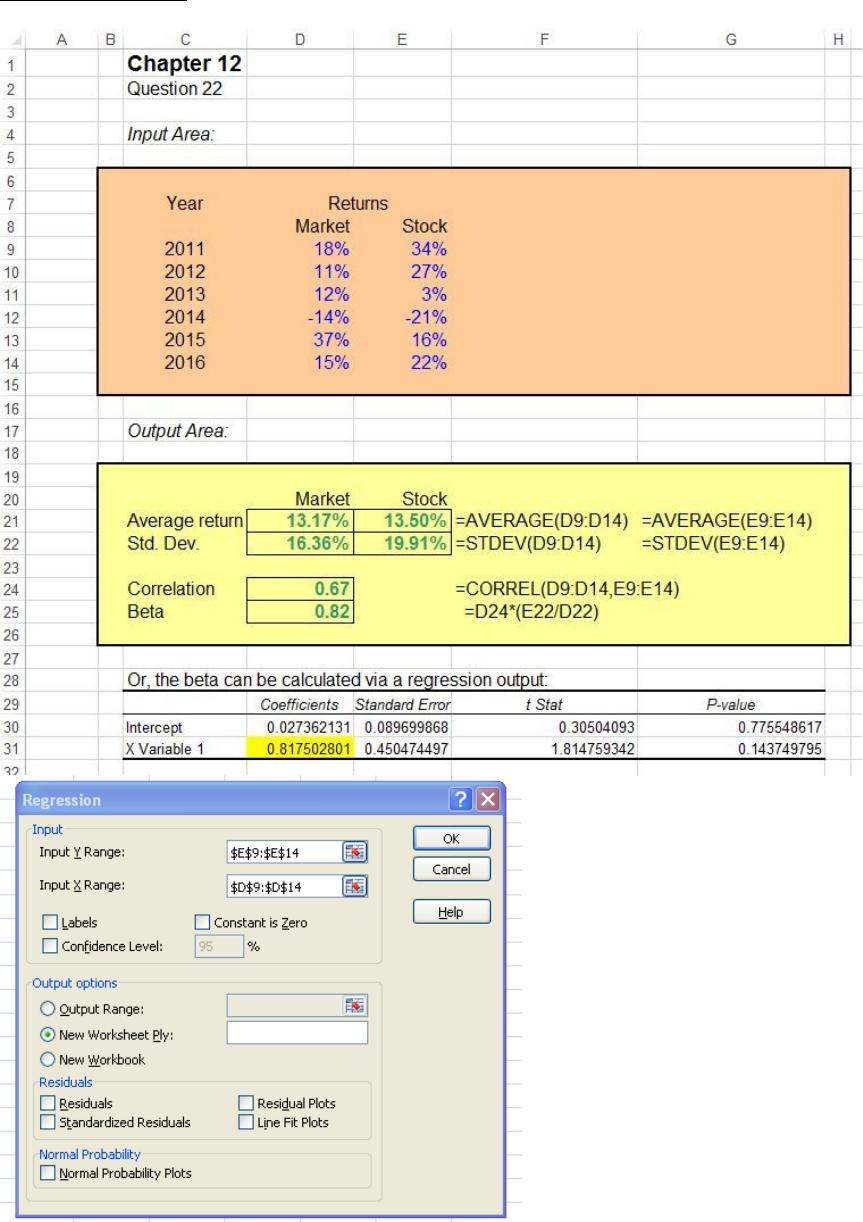Education.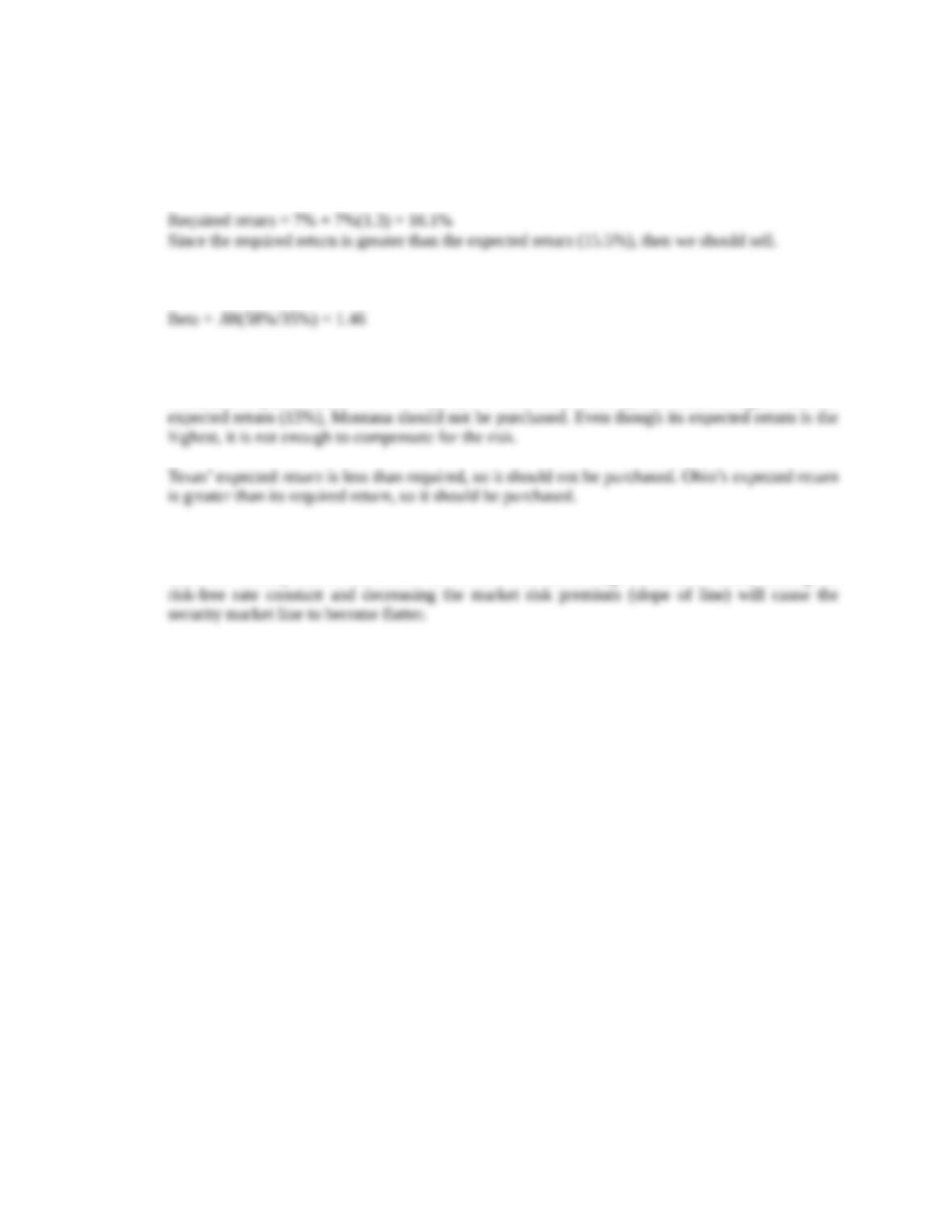CFA Exam Review by Kaplan Schweser
1. a
2. c
3. a
Montana’s required return = 7% + 7%(1.5) = 17.5%. Since this required return is higher than the
4. b
Since the security market line runs from the risk-free rate through the market return, holding the